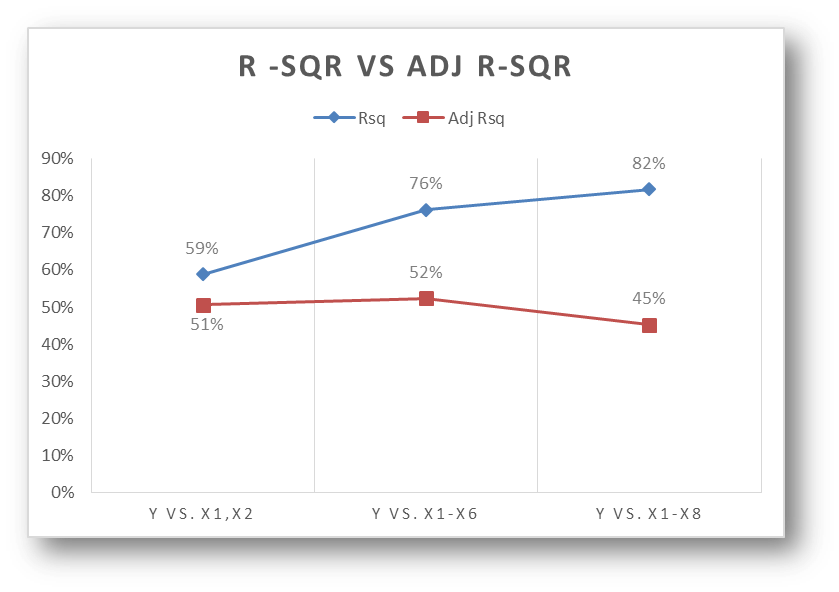• No products in the cart.

# 204.1.7 Adjusted R-squared in Python

##### R square for multiple variables in regression.

Link to the previous post : https://statinfer.com/204-1-6-multiple-regression-in-python/

• Is it good to have as many independent variables as possible? Nope
• R-square is deceptive. R-squared never decreases when a new X variable is added to the model – True?
• We need a better measure or an adjustment to the original R-squared formula.
• Its value depends on the number of explanatory variables
• It is usually written as• Very different fromwhen there are too many predictors and n is less

$\bar{R}^2 = R^2 – \frac{k-1}{n-k}(1-R^2)$

where n – number of observations k – number of parameters

R squared value increase if we increase the number of independent variables. Adjusted R-square increases only if a significant variable is added.  Look at this example. As we are adding new variables, R square increases, Adjusted R-square may not increase.

• Build a model to predict y using x1,x2 and x3. Note down R-Square and Adj R-Square values
• Build a model to predict y using x1,x2,x3,x4,x5 and x6. Note down R-Square and Adj R-Square values
• Build a model to predict y using x1,x2,x3,x4,x5,x6,x7 and x8. Note down R-Square and Adj R-Square values
In :
adj_sample=pd.read_csv("datasets\\Adjusted RSquare\\Adj_Sample.csv")

Out:
(12, 9)
In :
adj_sample.columns.values

Out:
array(['Y', 'x1', 'x2', 'x3', 'x4', 'x5', 'x6', 'x7', 'x8'], dtype=object)
In :
#Build a model to predict y using x1,x2 and x3. Note down R-Square and Adj R-Square values
from sklearn.linear_model import LinearRegression
lr = LinearRegression()

In :
import statsmodels.formula.api as sm
model = sm.ols(formula='Y ~ x1+x2+x3', data=adj_sample)
fitted1 = model.fit()
fitted1.summary()

C:\Anaconda3\lib\site-packages\scipy\stats\stats.py:1557: UserWarning: kurtosistest only valid for n>=20 ... continuing anyway, n=12
"anyway, n=%i" % int(n))

Out:
Dep. Variable: R-squared: Y 0.684 OLS 0.566 Least Squares 5.785 Wed, 27 Jul 2016 0.0211 11:48:28 -10.430 12 28.86 8 30.80 3 nonrobust
coef std err t P>|t| [95.0% Conf. Int.] -2.8798 1.163 -2.477 0.038 -5.561 -0.199 -0.4894 0.370 -1.324 0.222 -1.342 0.363 0.0029 0.001 2.586 0.032 0.000 0.005 0.4572 0.176 2.595 0.032 0.051 0.864
 Omnibus: Durbin-Watson: 1.113 1.978 0.573 0.763 -0.562 0.683 2.489 6000
In :
#Build a model to predict y using x1,x2,x3,x4,x5 and x6. Note down R-Square and Adj R-Square values

from sklearn.linear_model import LinearRegression
lr = LinearRegression()

In :
import statsmodels.formula.api as sm
model = sm.ols(formula='Y ~ x1+x2+x3+x4+x5+x6', data=adj_sample)
fitted2 = model.fit()
fitted2.summary()

C:\Anaconda3\lib\site-packages\scipy\stats\stats.py:1557: UserWarning: kurtosistest only valid for n>=20 ... continuing anyway, n=12
"anyway, n=%i" % int(n))

Out:
Dep. Variable: R-squared: Y 0.717 OLS 0.377 Least Squares 2.111 Wed, 27 Jul 2016 0.215 11:48:28 -9.7790 12 33.56 5 36.95 6 nonrobust
coef std err t P>|t| [95.0% Conf. Int.] -5.3751 4.687 -1.147 0.303 -17.423 6.673 -0.6697 0.537 -1.247 0.268 -2.050 0.711 0.0030 0.002 1.956 0.108 -0.001 0.007 0.5063 0.249 2.036 0.097 -0.133 1.146 0.0376 0.084 0.449 0.672 -0.178 0.253 0.0436 0.169 0.258 0.806 -0.390 0.478 0.0516 0.088 0.588 0.582 -0.174 0.277
 Omnibus: Durbin-Watson: 0.426 2.065 0.808 0.434 -0.347 0.805 2.378 19800
In :
from sklearn.linear_model import LinearRegression
lr = LinearRegression()

In :
import statsmodels.formula.api as sm
model = sm.ols(formula='Y ~ x1+x2+x3+x4+x5+x6+x7+x8', data=adj_sample)
fitted3 = model.fit()
fitted3.summary()

C:\Anaconda3\lib\site-packages\scipy\stats\stats.py:1557: UserWarning: kurtosistest only valid for n>=20 ... continuing anyway, n=12
"anyway, n=%i" % int(n))

Out:
Dep. Variable: R-squared: Y 0.805 OLS 0.285 Least Squares 1.549 Wed, 27 Jul 2016 0.393 11:48:28 -7.5390 12 33.08 3 37.44 8 nonrobust
coef std err t P>|t| [95.0% Conf. Int.] 17.0440 19.903 0.856 0.455 -46.297 80.385 -0.0956 0.761 -0.126 0.908 -2.519 2.328 0.0007 0.003 0.291 0.790 -0.007 0.009 0.5157 0.306 1.684 0.191 -0.459 1.490 0.0579 0.103 0.560 0.615 -0.271 0.387 0.0858 0.191 0.448 0.684 -0.524 0.695 -0.1747 0.220 -0.795 0.485 -0.874 0.525 -0.0324 0.153 -0.212 0.846 -0.519 0.455 -0.2321 0.207 -1.124 0.343 -0.890 0.425
 Omnibus: Durbin-Watson: 1.329 1.594 0.514 0.875 -0.339 0.646 1.863 78500
Model R2
Model1 0.684 0.566
Model2 0.717 0.377
Model3 0.805 0.285

We have built three models on Adj_sample data; model1, model2 and model3 with different number of variabes1) What does it indicate if R-square is very far away from Adj-R square?  An indication of too many variables/ Too many insignificant variables.   We may have to see the variable impact test and drop few independent variables from the model.

2) How do you use Adj-R square? Build a model, Calculate R-square is near to adjusted R-square. If not, use variable selection techniques to bring R square near to Adj- R square.  A difference of 2% between R square and Adj-R square is acceptable.

3) Is the only number of independent variables that make Adj-R Square down? No, if observe the formula carefully then we can see Adj-R square is influenced by k(number of variables) and n(number of observations) . If ‘k’ is high and ‘n’ is low then Adj-R Square will be very less.

Finally either reduce number of variables or increase the number of observations to bring Adj-R Square close to R Square

The next post is a practice session on multiple regression issues.
24th January 2018